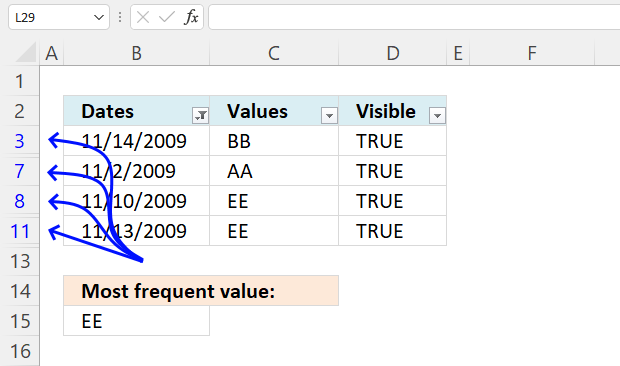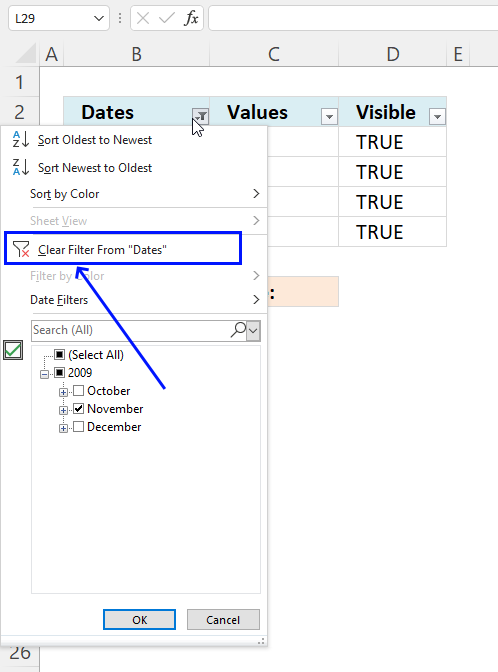Author: Oscar Cronquist Article last updated on October 12, 2022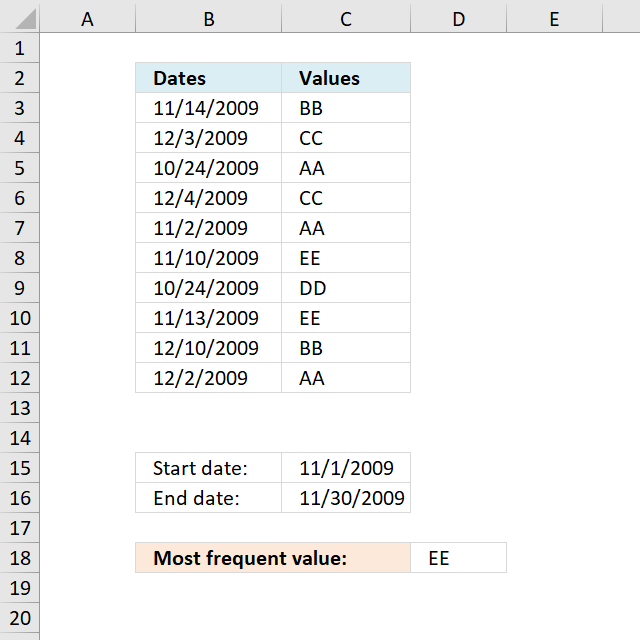I will in this article show you how to extract the most frequent value (text or number) between two dates in a column.

## 1. Most frequent value between two datesArray formula in D18:

=INDEX(\$C\$3:\$C\$12, MATCH(MODE.SNGL(IF((\$B\$3:\$B\$12<=\$C\$16)*(\$B\$3:\$B\$12>=\$C\$15), COUNTIF(\$C\$3:\$C\$12, "<"&\$C\$3:\$C\$12), "")), COUNTIF(\$C\$3:\$C\$12, "<"&\$C\$3:\$C\$12),0))

To enter an array formula, type the formula in a cell then press and hold CTRL + SHIFT simultaneously, now press Enter once. Release all keys.

The formula bar now shows the formula with a beginning and ending curly bracket telling you that you entered the formula successfully. Don't enter the curly brackets yourself.

### 1.1 Explaining the formula in cell D18

#### Step 1 - Calculate rank order if sorted

The COUNTIF function counts values based on a condition or criteria, the < less than sign makes the COUNTIF calculate a rank number if the list were sorted from A to Z.

COUNTIF(\$C\$3:\$C\$12,"<"&\$C\$3:\$C\$12)

becomes

COUNTIF({"BB"; "CC"; "AA"; "CC"; "AA"; "EE"; "DD"; "EE"; "BB"; "AA"},"<"&{"BB"; "CC"; "AA"; "CC"; "AA"; "EE"; "DD"; "EE"; "BB"; "AA"})

becomes

COUNTIF({"BB"; "CC"; "AA"; "CC"; "AA"; "EE"; "DD"; "EE"; "BB"; "AA"}, {"<BB";"<CC";"<AA";"<CC";"<AA";"<EE";"<DD";"<EE";"<BB";"<AA"})

and returns

{3;5;0;5;0;8;7;8;3;0}.

#### Step 2 - Check which values are in range

The IF function returns the rank number number based on a logical expression. It returns boolean value TRUE if the value is in the date range. If boolean value is FALSE the IF function returns "" (nothing).

IF((\$B\$3:\$B\$12<=\$C\$16)*(\$B\$3:\$B\$12>=\$C\$15),COUNTIF(\$C\$3:\$C\$12,"<"&\$C\$3:\$C\$12),"")

becomes

IF({1;0;0;0;1;1;0;1;0;0},COUNTIF(\$C\$3:\$C\$12,"<"&\$C\$3:\$C\$12),"")

becomes

IF({1;0;0;0;1;1;0;1;0;0}, {3; 5; 0; 5; 0; 8; 7; 8; 3; 0},"")

and returns

{3; ""; ""; ""; 0; 8; ""; 8; ""; ""}.

#### Step 3 - Calculate the most frequent number

The MODE.SNGL function returns the most frequent number in a cell range or array.

MODE.SNGL(IF((\$B\$3:\$B\$12<=\$C\$16)*(\$B\$3:\$B\$12>=\$C\$15),COUNTIF(\$C\$3:\$C\$12,"<"&\$C\$3:\$C\$12),""))

becomes

MODE.SNGL({3; ""; ""; ""; 0; 8; ""; 8; ""; ""})

and returns 8.

#### Step 4 - Find position of most frequent number in array

The MATCH function finds the relative position of a value in an array or cell range.

MATCH(MODE.SNGL(IF((\$B\$3:\$B\$12<=\$C\$16)*(\$B\$3:\$B\$12>=\$C\$15), COUNTIF(\$C\$3:\$C\$12, "<"&\$C\$3:\$C\$12), "")), COUNTIF(\$C\$3:\$C\$12, "<"&\$C\$3:\$C\$12),0)

becomes

MATCH(8, COUNTIF(\$C\$3:\$C\$12, "<"&\$C\$3:\$C\$12),0)

becomes

MATCH(8, {3; 5; 0; 5; 0; 8; 7; 8; 3; 0},0)

and returns 6.

#### Step 5 - Return value

The INDEX function returns a value based on row number (and column number if needed)

INDEX(\$C\$3:\$C\$12, MATCH(MODE.SNGL(IF((\$B\$3:\$B\$12<=\$C\$16)*(\$B\$3:\$B\$12>=\$C\$15), COUNTIF(\$C\$3:\$C\$12, "<"&\$C\$3:\$C\$12), "")), COUNTIF(\$C\$3:\$C\$12, "<"&\$C\$3:\$C\$12),0))

becomes

INDEX(\$C\$3:\$C\$12, 6)

and returns "EE" in cell D18.

Get Excel *.xlsx file

Most common value between two dates.xlsx

## 2. Most frequent value between two dates - Autofilter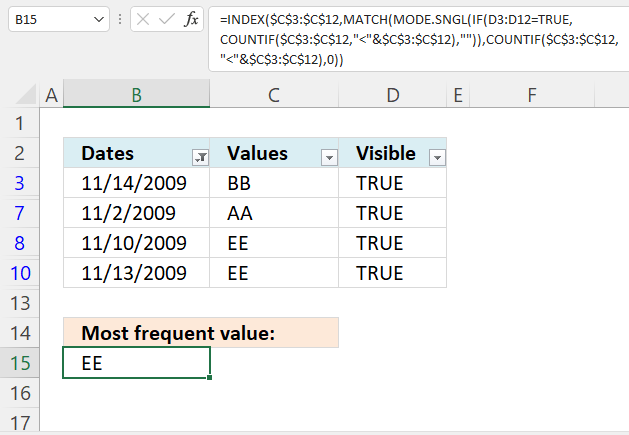This example demonstrates how to identify the most repeated value in a filtered data set using the Autofilter feature and two formulas.

Formula in cell B15:

=INDEX(\$C\$3:\$C\$12, MATCH(MODE.SNGL(IF(D3:D12=1, COUNTIF(\$C\$3:\$C\$12, "<"&\$C\$3:\$C\$12), "")), COUNTIF(\$C\$3:\$C\$12, "<"&\$C\$3:\$C\$12), 0))

Formula in cell D3:

=SUBTOTAL(3,C3)

Copy cell D3 and paste to the cells below.

### 2.1 Explaining formula in cell D3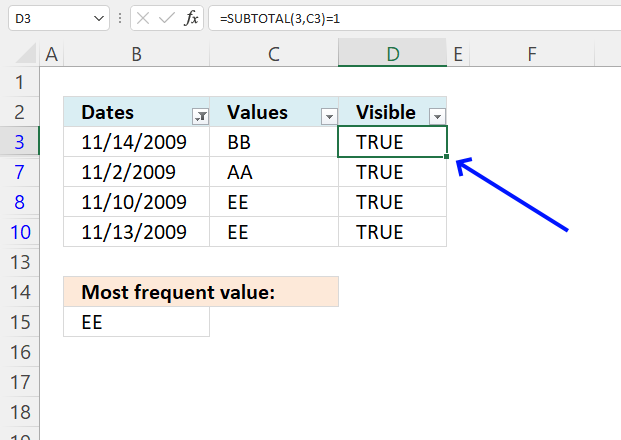#### Step 1 - Populate arguments

The SUBTOTAL function returns a subtotal from a list or database, you can choose from a variety of arguments that determine what you want the function to do.

Function syntax: SUBTOTAL(function_num, ref1, ...)

SUBTOTAL(function_num, ref1, ...)

becomes

SUBTOTAL(3,C3)

SUBTOTAL(3,C3)

becomes

SUBTOTAL(3,"BB")

and returns 1.

#### Step 3 - Check if the value is equal to 1

The equal sign is a logical operator, it compares value to value. The result is a boolean value TRUE or FALSE.

SUBTOTAL(3, C3)=1

becomes

1=1

and returns

TRUE.

### 2.2 Explaining formula in cell B15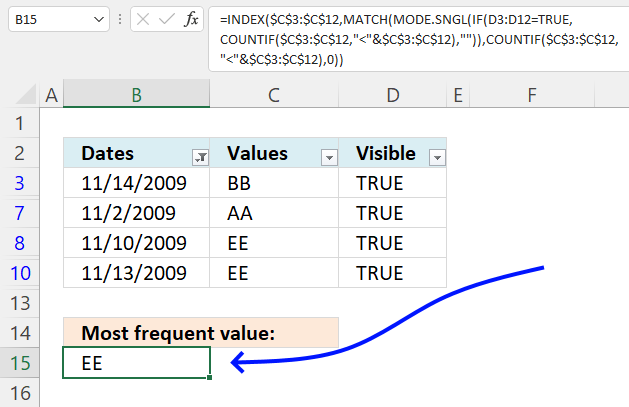#### Step 1 - Check which rows are visible

The equal sign is a logical operator, it compares value to value. The result is a boolean value TRUE or FALSE.

D3:D12=TRUE

{TRUE;0;0;0;TRUE;TRUE;0;0;TRUE;FALSE} = TRUE

and returns

{TRUE; FALSE; FALSE; FALSE; TRUE; TRUE; FALSE; FALSE; TRUE; FALSE}.

#### Step 2 - Convert text values to unique numbers

The COUNTIF function calculates the number of cells that is equal to a condition.

Function syntax: COUNTIF(range, criteria)

COUNTIF(\$C\$3:\$C\$12, "<"&\$C\$3:\$C\$12)

becomes

COUNTIF({"BB"; "CC"; "AA"; "CC"; "AA"; "EE"; "AA"; "DD"; "EE"; "BB"}, "<"&{"BB"; "CC"; "AA"; "CC"; "AA"; "EE"; "AA"; "DD"; "EE"; "BB"})

and returns

{3; 5; 0; 5; 0; 8; 0; 7; 8; 3}.

#### Step 3 - Replace visible values with a unique number

The IF function returns one value if the logical test is TRUE and another value if the logical test is FALSE.

Function syntax: IF(logical_test, [value_if_true], [value_if_false])

IF(D3:D12=1, COUNTIF(\$C\$3:\$C\$12, "<"&\$C\$3:\$C\$12), "")

becomes

IF(TRUE; FALSE; FALSE; FALSE; TRUE; TRUE; FALSE; FALSE; TRUE; FALSE}, {3; 5; 0; 5; 0; 8; 0; 7; 8; 3}, "")

and returns

{3; ""; ""; ""; 0; 8; ""; ""; 8; ""}

#### Step 4 - Find the most repeated number

The MODE.SNGL function calculates the most frequent value in an array or range of data.

Function syntax: MODE.SNGL(number1,[number2],...)

MODE.SNGL(IF(D3:D12=1, COUNTIF(\$C\$3:\$C\$12, "<"&\$C\$3:\$C\$12), ""))

becomes

MODE.SNGL({3; ""; ""; ""; 0; 8; ""; ""; 8; ""})

and returns 8.

#### Step 5 - Find the relative position of the most repeated number

The MATCH function returns the relative position of an item in an array that matches a specified value in a specific order.

Function syntax: MATCH(lookup_value, lookup_array, [match_type])

MATCH(MODE.SNGL(IF(D3:D12=1, COUNTIF(\$C\$3:\$C\$12, "<"&\$C\$3:\$C\$12), "")), COUNTIF(\$C\$3:\$C\$12, "<"&\$C\$3:\$C\$12), 0)

becomes

MATCH(8, {3; 5; 0; 5; 0; 8; 0; 7; 8; 3}, 0)

and returns

6.

#### Step 6 - Get the corresponding value in \$C\$3:\$C\$12

The INDEX function returns a value or reference from a cell range or array, you specify which value based on a row and column number.

Function syntax: INDEX(array, [row_num], [column_num])

INDEX(\$C\$3:\$C\$12, MATCH(MODE.SNGL(IF(D3:D12=1, COUNTIF(\$C\$3:\$C\$12, "<"&\$C\$3:\$C\$12), "")), COUNTIF(\$C\$3:\$C\$12, "<"&\$C\$3:\$C\$12), 0))

becomes

INDEX(\$C\$3:\$C\$12, 6)

becomes

INDEX({"BB"; "CC"; "AA"; "CC"; "AA"; "EE"; "AA"; "DD"; "EE"; "BB"}, 6)

and returns

"EE".

### 2.3 How to enable the Autofilter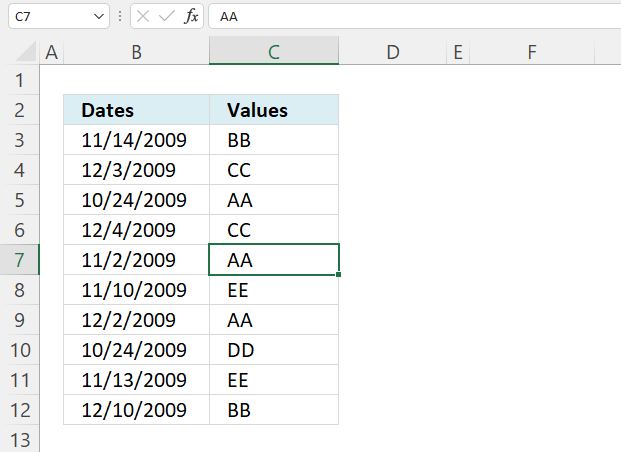How do I enable the autofilter for a given data set (shortcut keys)?

1. Select any cell in the data set.
2. Press and hold CTRL and Shift keys.
3. Press L once.
4. Release all keys.

How do I enable the autofilter for a given data set (button on the ribbon)?

1. Select any cell in the data set.
2. Go to tab "Data".
3. Press with left mouse button on the "Filter" button.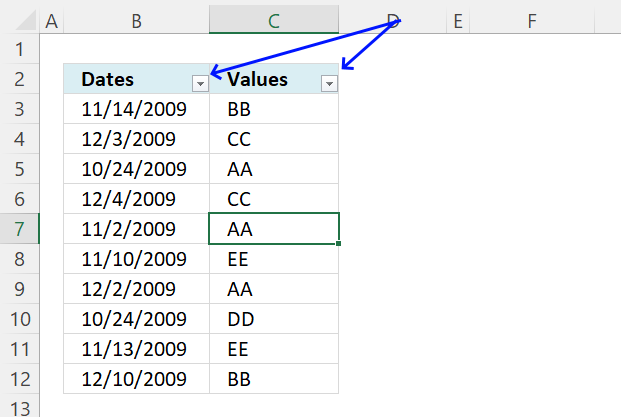How do I know autofilter is enabled?

The column header names have a small button each containing an arrow, see the image above.

### 2.4 Enter the formula in cell D3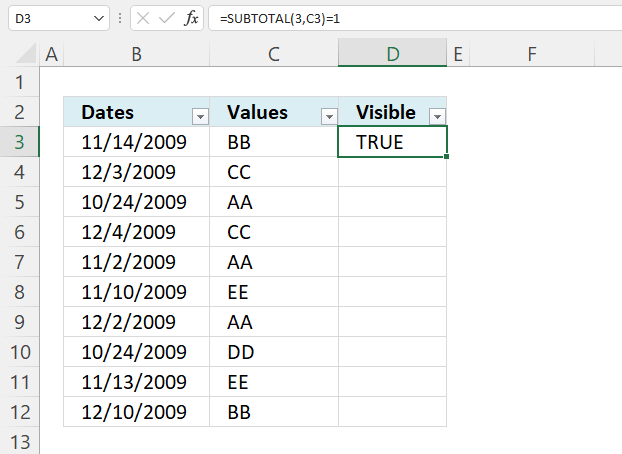Copy cell D3 and paste to the cells below.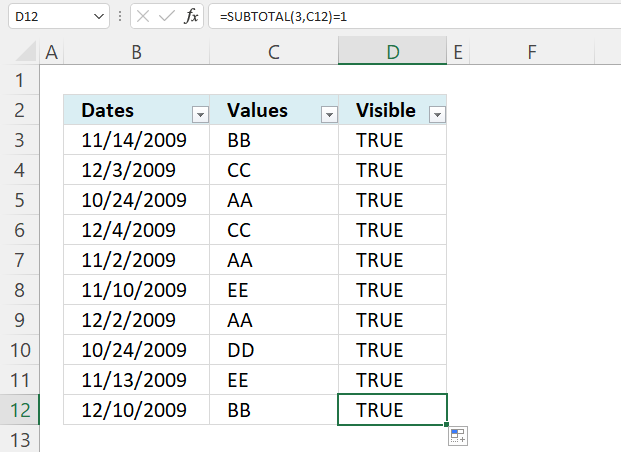Make sure the new column header has the autofilter arrow. If not, disable the autofilter and then enable it again.

### 2.5 How to apply a condition to the Autofilter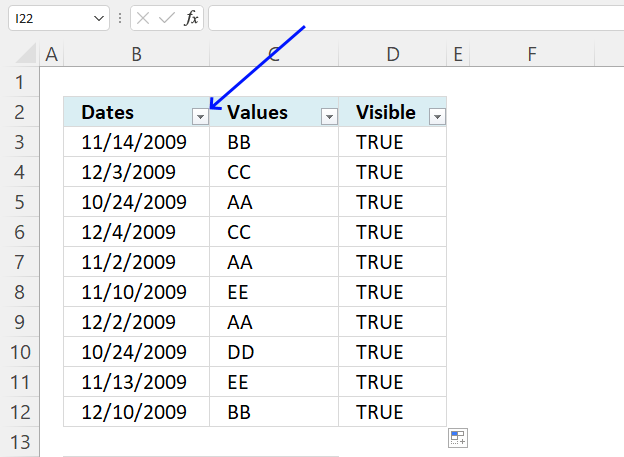1. Press with mouse on a button next to a column you want to filter.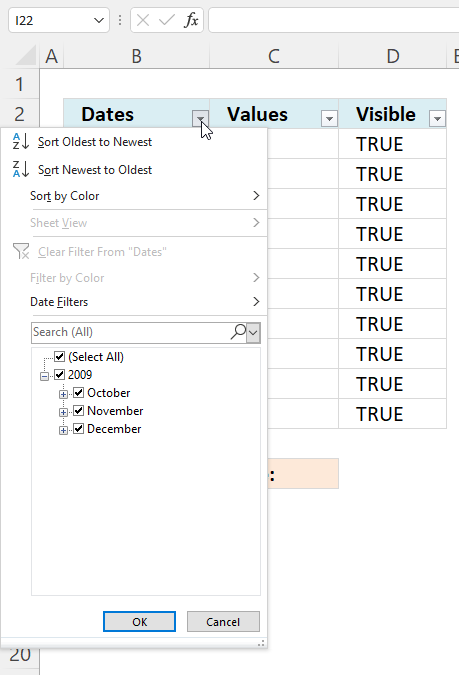2. A popup menu appears. Deselect all check boxes except "November".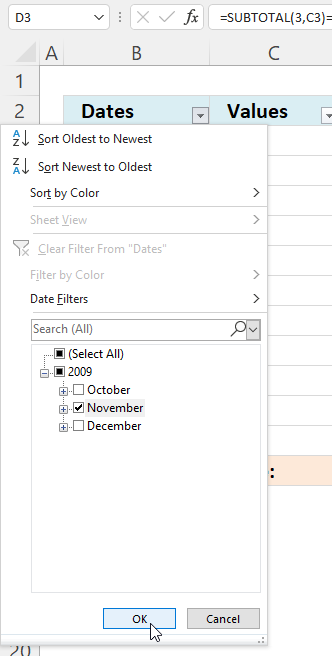3. Press with left mouse button on "OK" button.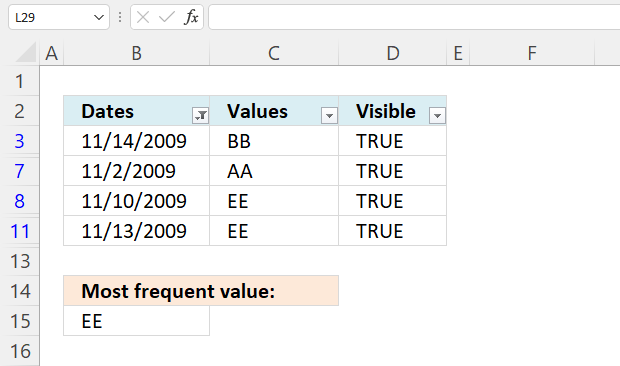### 2.6 How to know if a data set is filtered - Autofilter?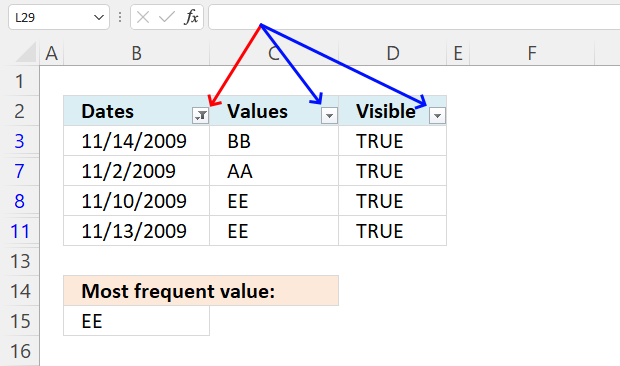There are two ways you can see if a data set is filtered, the first one is the button.

The button next to column header name "Date" has changed from an arrow to an icon that tells you the data is filtered.

The second one is the row color, filtered data has blue row numbers.